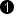## Operator RND

The operator RND performs a right shift with rounding and clipping to the output bit width. The number of bits to round is specified with parameter bits2Round.

Round half away from zero is the implemented rounding method. Color components are rounded separately.

Examples

• Input = 4 Bit unsigned, Input Value = 7, bits2Round = 2

Output = 2 Bit unsigned, Output Value = 2

• Input = 5 Bit signed, Input Value = -13, bits2Round = 2

Output = 3 Bit signed, Output Value = -3

• Input = 4 Bit unsigned, Input Value = 15, bits2Round = 2

Output = 2 Bit unsigned, Output Value = 3 Note: The output has been clipped to the maximum possible value.

### I/O Properties

Property Value
Operator Type O
Input Link I, data input
Output Link O, data output

### Supported Link Format

Bit Width [1, 64] unsigned, [2, 64] signed autoArithmetic {unsigned, signed} as I
Parallelism any as I
Kernel Columns any as I
Kernel Rows any as I
Img Protocol {VALT_IMAGE2D, VALT_LINE1D, VALT_PIXEL0D} as I
Color Format any as I
Color Flavor any as I
Max. Img Width any as I
Max. Img Height any as I
 The bit width at the output is the input bit width minus parameter value of bits2round.

### Parameters

bits2round
Type static parameter
Default 0
Range

[0, BitWidth(I)-1] for unsigned

(BitWidth(I) - bits2Round > 1) for signed

This parameter defines by how many bits the input is shifted to the right.

### Examples of Use

The use of operator RND is shown in the following examples: